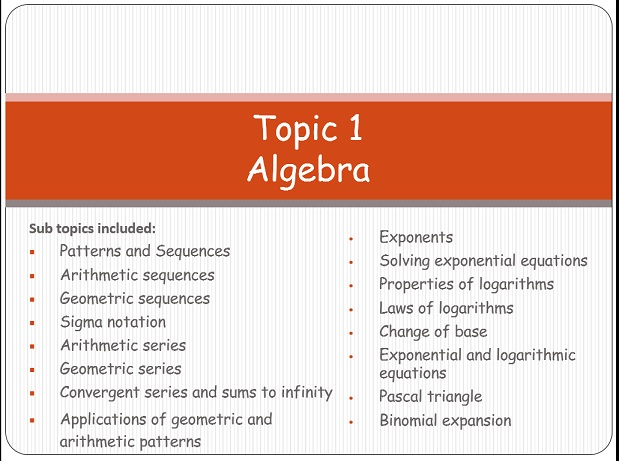Refer a friend and get % off! They'll get % off too.# Bundle Topic 1 - Algebra SL

This is a ZIP file including all the Power point presentations for the Topic 1 - Algebra based on the  IB Mathematics SL Syllabus. The sub topics are:

Patterns and Sequences
Arithmetic sequences
Geometric sequences
Sigma notation
Arithmetic series
Geometric series
Convergent series and sums to infinity
Applications of geometric and arithmetic patterns

Exponents
Solving exponential equations
Properties of logarithms
Laws of logarithms
Change of base
Exponential and logarithmic equations
Pascal triangle
Binomial expansion

You will get a ZIP (16MB) file

£ 45.00

£ 45.00

Discount has been applied.

orAdding ...